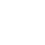# How to add a variable with input-box?

Twine 2.3.14 Harlowe 3.2.2

Hello,
I’m sorry if you already discussed this. I searched the discussion and didn’t find it.
I would like to involve the player more in the game and I thought of this possibility.
At the beginning of the game, the player has a certain number of points, which he loses in the game, but also gains.
It occurred to me that he would roll the dice and add the points himself.
During the game you may get another chance to increase your points in the same way.
I bother with macros, but unfortunately to no avail.
Can you advise me if this can be applied at all?
My definitely bad Macro code looks like this:

``````(random: 1.30)
(input-box: bind \$points, "X", 1)
``````

Jiří Škrába

There is a syntax error in your `(random: 1.30)` macro call, the separator between the two numeric arguments being passed to the macro should be a coma, and not a full-stop.

``````(random: 1, 30)
``````

And if you want to be able to use the generated random number later on in that Passage then you need to store that number in a variable.

``````(set: _roll to (random: 1, 30))
``````

You would use code like the following to increase a numerical value stored in a variable (like `\$points`) by another numerical value…

``````(set: \$points to it + 5)
``````

…and you use a similar syntax if the 2nd numerical value is also stored in a variable.

``````(set: _amount to 5)

(set: \$points to it + _amount)
``````

Unfortunately it is unclear from your question and the supplied example what the exact relationship between the `(random:)` macro call and the `(input-box:)` macro call is. If I’ve guessed right then I believe it was something like…

``````(set: _amount to (random: 1, 30))

(input-box: bind _amount, "X", 1)
(set: \$points to it + _amount)
]
``````

…although I am a little confused why you’re allowing the end-user to edit/change the random number you generated.

Hello,

thanks for the code but i still have problem.

I will explain:

I create a game based on a scenario like Game Book.

There, the player rolls the dice and writes the result in a notebook. During the game, he loses and gains points.

I copied your code and put it into the game.

Everything is OK until I write the generated cube number in (input-box:).

This message will then appear.

The number 5 isn’t the same type of data as the string “28”I am a beginner and still in old ageSo the codes creep into me slowly.

I know I can give a player points myself (set: \$points to 30). Then read them (set: \$points to it - 1).

I would like the player to be more active and think that he controls the game himself.

Notebook players will always show in the passage where there is no variable.

The dice are an important element for the game.

Jiří ŠkrábaBy default the `(input-box:)` macro only allows the inputting of String values.

The number 5 isn’t the same type of data as the string “28”

The error message you received when comparing the entered “28” String value with the Number 5 value, is just telling you that the two values have different data types so they can’t be compared.

If you want to treat the entered “28” String value as a Number then you need to change the value’s data-type using the `(num:)` macro.
(note: you didn’t supply an example of your code so I don’t know how you are comparing the entered value, so I have crafted the following as a very basic example of how to use the `(num:)` macro)

``````(input-box: bind _amount, "X", 1)

(set: _amount to (num: _amount))
(if: _amount is 5)[
(goto: "Other Passage")
]
]
``````

Hello,

many thanksThat’s exactly it.My code now looks like this.

Maybe there are Macros that don’t belong there, but it works.

(random: 1.30)]

(set: \$points to 0)

(set: _roll to (random: 1, 30))

(set: \$amount to 0)

(set: \$amount to (random: 1, 30))

(input-box: bind \$amount, “X”, 1)

(set: \$amount to (num: \$amount))

(set: \$points to it + \$amount)

(if: \$amount is 0) [

(goto: “Other Passage”)]]

You are the best and thank you very much

Jiří Škrába